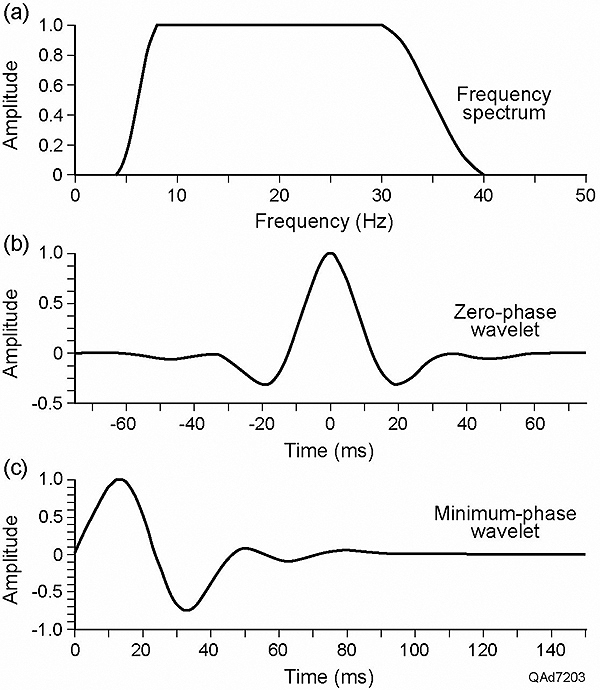--> Figure 2. (a) Amplitude spectrum of the Ormsby wavelet used in modeling. The four corner frequencies are 4, 8, 30, and 40 Hz; (b) The corresponding zero-phase wavelet; (c) The corresponding minimum-phase wavelet.Figure 2. (a) Amplitude spectrum of the Ormsby wavelet used in modeling. The four corner frequencies are 4, 8, 30, and 40 Hz; (b) The corresponding zero-phase wavelet; (c) The corresponding minimum-phase wavelet.